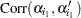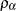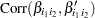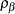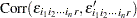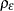Analysis of Covariation

When you specify more than one dependent variable, the NESTED procedure produces a descriptive analysis of the covariance between each pair of dependent variables in addition to a separate analysis of variance for each variable. The analysis of covariation is computed under the basic random-effects model for each pair of dependent variables: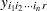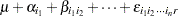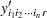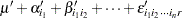where the notation is the same as that used in the preceding general random-effects model.

There is an additional assumption that all the random effects in the two models are mutually uncorrelated except for corresponding effects, for which